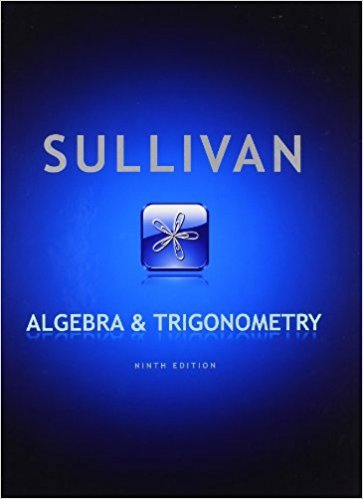×
×

# Solutions for Chapter 11.3: Algebra and Trigonometry 9th Edition## Full solutions for Algebra and Trigonometry | 9th Edition

ISBN: 9780321716569Solutions for Chapter 11.3

Solutions for Chapter 11.3
4 5 0 366 Reviews
24
1
##### ISBN: 9780321716569

Algebra and Trigonometry was written by and is associated to the ISBN: 9780321716569. This textbook survival guide was created for the textbook: Algebra and Trigonometry, edition: 9. Since 85 problems in chapter 11.3 have been answered, more than 58162 students have viewed full step-by-step solutions from this chapter. Chapter 11.3 includes 85 full step-by-step solutions. This expansive textbook survival guide covers the following chapters and their solutions.

Key Math Terms and definitions covered in this textbook
• Change of basis matrix M.

The old basis vectors v j are combinations L mij Wi of the new basis vectors. The coordinates of CI VI + ... + cnvn = dl wI + ... + dn Wn are related by d = M c. (For n = 2 set VI = mll WI +m21 W2, V2 = m12WI +m22w2.)

• Four Fundamental Subspaces C (A), N (A), C (AT), N (AT).

Use AT for complex A.

• Graph G.

Set of n nodes connected pairwise by m edges. A complete graph has all n(n - 1)/2 edges between nodes. A tree has only n - 1 edges and no closed loops.

• Hessenberg matrix H.

Triangular matrix with one extra nonzero adjacent diagonal.

• Kirchhoff's Laws.

Current Law: net current (in minus out) is zero at each node. Voltage Law: Potential differences (voltage drops) add to zero around any closed loop.

• Left inverse A+.

If A has full column rank n, then A+ = (AT A)-I AT has A+ A = In.

• Left nullspace N (AT).

Nullspace of AT = "left nullspace" of A because y T A = OT.

• Matrix multiplication AB.

The i, j entry of AB is (row i of A)·(column j of B) = L aikbkj. By columns: Column j of AB = A times column j of B. By rows: row i of A multiplies B. Columns times rows: AB = sum of (column k)(row k). All these equivalent definitions come from the rule that A B times x equals A times B x .

• Multiplication Ax

= Xl (column 1) + ... + xn(column n) = combination of columns.

• Normal matrix.

If N NT = NT N, then N has orthonormal (complex) eigenvectors.

• Nullspace matrix N.

The columns of N are the n - r special solutions to As = O.

• Orthogonal matrix Q.

Square matrix with orthonormal columns, so QT = Q-l. Preserves length and angles, IIQxll = IIxll and (QX)T(Qy) = xTy. AlllAI = 1, with orthogonal eigenvectors. Examples: Rotation, reflection, permutation.

• Permutation matrix P.

There are n! orders of 1, ... , n. The n! P 's have the rows of I in those orders. P A puts the rows of A in the same order. P is even or odd (det P = 1 or -1) based on the number of row exchanges to reach I.

• Pivot.

The diagonal entry (first nonzero) at the time when a row is used in elimination.

• Plane (or hyperplane) in Rn.

Vectors x with aT x = O. Plane is perpendicular to a =1= O.

• Rank r (A)

= number of pivots = dimension of column space = dimension of row space.

• Row space C (AT) = all combinations of rows of A.

Column vectors by convention.

• Saddle point of I(x}, ... ,xn ).

A point where the first derivatives of I are zero and the second derivative matrix (a2 II aXi ax j = Hessian matrix) is indefinite.

• Solvable system Ax = b.

The right side b is in the column space of A.

• Unitary matrix UH = U T = U-I.

Orthonormal columns (complex analog of Q).

×